IMO Shortlist 2014 problem A3

Kvaliteta:
Avg: 0,0
Težina:
Avg: 7,0

For a sequence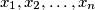$x_1, x_2, \ldots, x_n$ of real numbers, we define its price as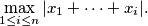Given$n$ real numbers, Dave and George want to arrange them into a sequence with a low price. Diligent Dave checks all possible ways and finds the minimum possible price$D$. Greedy George, on the other hand, chooses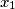$x_1$ such that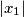$|x_1|$ is as small as possible; among the remaining numbers, he chooses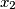$x_2$ such that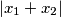$|x_1 + x_2|$ is as small as possible, and so on. Thus, in the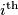$i^{\text{th}}$ step he chooses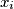$x_i$ among the remaining numbers so as to minimise the value of$|x_1 + x_2 + \dots + x_i|$. In each step, if several numbers provide the same value, George chooses one at random. Finally he gets a sequence with price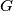$G$.

Find the least possible constant$c$ such that for every positive integer$n$, for every collection of$n$ real numbers, and for every possible sequence that George might obtain, the resulting values satisfy the inequality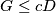$G \leq c D$.

(Georgia)

Izvor: https://www.imo-official.org/problems/IMO2014SL.pdf